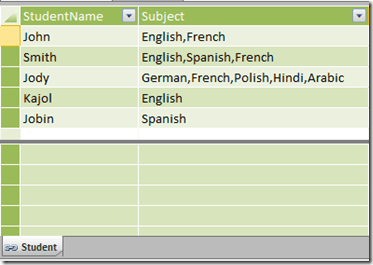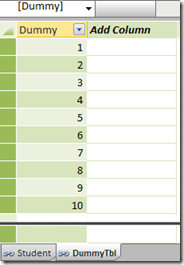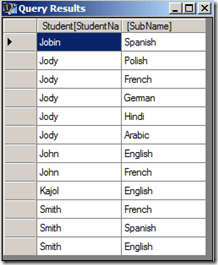# Split a Delimited Row into Multiple Rows using DAX Queries

Recently, I got a question from one of my readers on whether there is a way to split a single delimited row into multiple rows using DAX. I had kind of demonstrated the opposite to Group Multiple Rows to Single Delimited Row in PowerPivot here and this was another interesting challenge. Read on for the solution.Suppose there is a table which has two columns – StudentName and Subject.The end result should beSolution

We will be using DAX queries to generate a table that will have the desired output. For this demonstration, I will be creating a PowerPivot model and running DAX queries in DAX Studio on top of the Excel PowerPivot model.

1) Create a linked table for the Student.2) Create a table called DummyTbl which is just a list of running numbers from 1 till n. The value of n should be equal to the max number of subjects that a student can have. In this demo, I am assuming that a student can have a max of 10 subjects, so my Dummy table will consist of numbers 1 – 10.3) Now let us create the DAX queries in DAX Studio. First, we will create a computed column called SubCnt which will give the number of subjects for each row.

EVALUATE
SUMMARIZE (
Student,
[StudentName],
[Subject],
“SubCnt”,
1 + Len ( [Subject] ) – Len ( Substitute ( [Subject], “,”, “” ) )
)4) The only way we can increase the number of rows of a table is by using the function CrossJoin. So let us CrossJoin the Student table with the DummyTbl.

EVALUATE
Crossjoin (
SUMMARIZE (
Student,
[StudentName],
[Subject],
“SubCnt”,
1 + Len ( [Subject] ) – Len ( Substitute ( [Subject], “,”, “” ) )
),
DummyTbl
)5) Now filter the resultant table where SubCnt is less than or equal to Dummy column. Now we have got the exact number of rows needed for the end result.

EVALUATE
Filter (
Crossjoin (
SUMMARIZE (
Student,
[StudentName],
[Subject],
“SubCnt”,
1 + Len ( [Subject] ) – Len ( Substitute ( [Subject], “,”, “” ) )
),
DummyTbl
),
DummyTbl[Dummy] <= [SubCnt]
)6) The only thing left is to split the delimited subjects to the single subject for each row. And the only way to split delimited values is to use the PATHITEM function. To use the PATHITEM function, we should substitute the commas (,) with the pipe (|) symbol.

EVALUATE
FILTER (
CROSSJOIN (
SUMMARIZE (
Student,
[StudentName],
[Subject],
“SubCnt”,
1 + Len ( [Subject] ) – Len ( Substitute ( [Subject], “,”, “” ) )
),
DummyTbl
),
DummyTbl[Dummy] <= [SubCnt]
),
“SubName”,
PATHITEM (
SUBSTITUTE ( Student[Subject], “,”, “|” ),
DummyTbl[Dummy]
)
)7) Now all we need to do is to select the two required columns.

EVALUATE
SUMMARIZE (
FILTER (
CROSSJOIN (
SUMMARIZE (
Student,
[StudentName],
[Subject],
“SubCnt”,
1 + Len ( [Subject] ) – Len ( Substitute ( [Subject], “,”, “” ) )
),
DummyTbl
),
DummyTbl[Dummy] <= [SubCnt]
),
“SubName”,
pathitem (
Substitute ( Student[Subject], “,”, “|” ),
DummyTbl[Dummy]
)
),
[StudentName],
[SubName]
)
ORDER BY [StudentName]Hopefully this post will help you when you encounter a similar situation!### Posted by SQLJasonLeonid Ore

Very useful technique. I have only one question: how can I bring the result back to powerpivot model?David Cor

You have to create a link back table to bring it back into the data model.Nick

Thanks for this useful technique. Can this be done in ‘native’ DAX? I have an application where I can’t use DAX Studio. Thanks.Marco De Angelis

Brilliant.

Thank for this great workThank you very much for this!
I have been searching for a solution for so long and you finally helped me to solve it.ukeer

you can replace the dummytable with GENERATESERIES, Like so:

= FILTER(
CROSSJOIN(
SUMMARIZE(
‘TableName’;
[Column1]; [Column2];
“PathLength”; PATHLENGTH([Column1])
);
GENERATESERIES(1; 25)
);
[PathLength] >= [Value]
)Cosmin

exactly what i needed!

Thanks a lot!
CosminCosmin